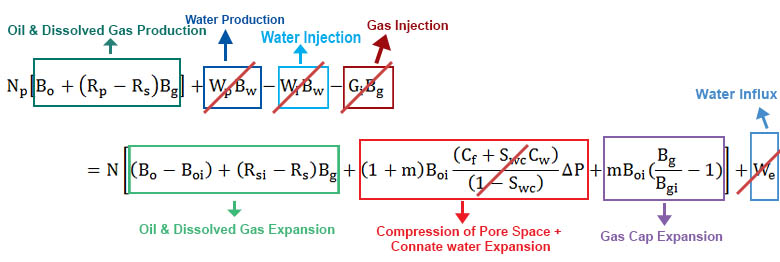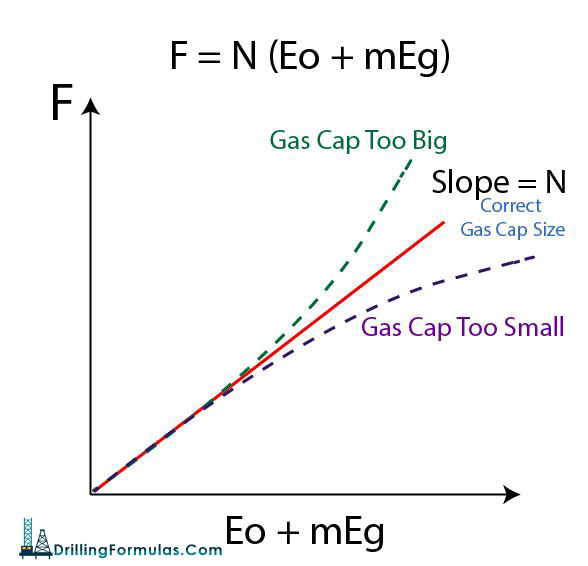# Material Balance for Gas Cap Drive MechanismFigure 1 – Full Material Balance Equation

Assumptions

• No water production
• No water injection
• No gas injection
• No water influx
• Neglect formation and connate water compressibility (Cf and Cw have little effect for a gas cap drive mechanism.)Figure 2 – Material Balance Equation with Assumption for a Gas Cap Drive Mechanism

The material balance shown in Figure 2 can be simplified like this:For a gas cap drive, oil and gas production is equal to oil + dissolved gas expansion pus gas cap expansion.

This can be described in a simple equation (Havlena + Odeh (1963)) like this:

# F = N (Eo + m Eg)

Where;

F = Net fluid production

N = Oil in place

Eo = oil expansion term

m = initial gas cap volume / initial oil volume (rb/rb)

Eg = gas expansion term

The plot is constructed between F and (Eo+mEg).Figure 3 – Graphic Solution for Gas Cap Drive Mechanism

If a plot shows a straight line slope, it means that gas cap volume is correct. Any deviation from a straight line indicates either a too small or too big gas cap size as shown in Figure 3.

Nomenclatures

N = oil initially in place (STOIIP) in reservoir (stb)

Np = cumulative oil production (stb)

Boi = oil volume factor at initial reservoir pressure (rb/stb)

Bo = oil volume factor at current reservoir pressure (rb/stb)

Rsi = solution GOR at initial reservoir pressure (scf/stb)

Rs = solution GOR at current reservoir pressure (scf/stb)

Rp = cumulative produced gas oil ratio (scf/stb)

G = gas volume initially in place (GIIP) in reservoir (scf)

m = ratio of initial gas cap volume to initial oil volume (rb/rb)

Bgi = gas volume factor at initial reservoir pressure (rb/scf)

Bg = gas volume factor at current reservoir pressure (rb/scf)

Swc = connate water saturation (fraction or %)

Cw = water compressibility (1/psi)

Cf = formation (rock) compressibility (1/psi)

Wp = cumulative water production (stb)

We= cumulative water influx from aquifer (rb)

Bw = water volume factor at initial reservoir pressure (rb/stb)

Wi = cumulative water injection (stb)

Gi = cumulative gas injection (scf)

Gp = cumulative gas production (scf)

Eg = gas expansion term (rb/stb)

Eo = oil expansion term (rb/stb)

Efw = formation and connate water expansion term (rb/stb)

References

Abhijit Y. Dandekar, 2013. Petroleum Reservoir Rock and Fluid Properties, Second Edition. 2 Edition. CRC Press.

L.P. Dake, 1983. Fundamentals of Reservoir Engineering, Volume 8 (Developments in Petroleum Science). New impression Edition. Elsevier Science.

Tarek Ahmed PhD PE, 2011. Advanced Reservoir Management and Engineering, Second Edition. 2 Edition. Gulf Professional Publishing.

Share the joy
Tagged , , , , , . Bookmark the permalink.